# CAD: Linear Algebra and the Synthetic Camera

An obvious requirement, in computer graphics, is to be able to view an object from many different points of view. This is accomplished by transforming the object with respect to a fix coordinate system, often referred to as the world coordinate system. Another approach is to define a viewing coordinate system that is free to move around in space. Think of a camera, fix in this system and pointing in the direction along the n-axis of the viewing coordinate system, defining the synthetic camera viewing direction.

## Using the Synthetic Camera

Just as a photographer strategically places their camera for different view, the synthetic camera can be used for framing a scene similar to looking through a camera's viewfinder. The portion of the camera's field of view that is used for viewing a scene is called the production frame. The production frame is similar to a real-world camera's viewfinder. The production frame is located in the uv-plane. The uvn-system is a movable system of coordinates. Fixed in the uvn-system is the production frame along with the camera positioned at the center of projection (COP). The eye or viewing point is located at the cop and defined in uvn-coordinates as the vector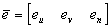.

The origin of the uvn-system or view reference point (VRP) is located at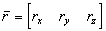in the world coordinate system. The view plane normal (VPN) specifies the n-axis of the uvn-system. The vpn is always normal to the viewing plane, and is represented by the unit vector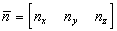as defined in world coordinates (xyz-system).

The "up" vector, also called the view up vector (VUP), is defined in the world coordinate system. The "up" vector is used to align the v-axis in the uvn-system. The direction "up" is usually defined in the same sense as "gravity". This gives a realistic view of an object, i.e. a viewer will have a sense of flying upside down in an aircraft, if a building is viewed as pointing downwards.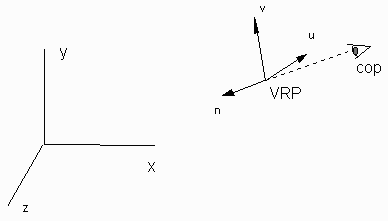The uvn-system is a left handed system. For most applications, the eye is located on the negative n-axis. The positive n-axis is directed away from the eye towards the object you want to "point at". The position of the object is fixed in the world coordinates.

## Example:

An object is located at the origin of the world coordinates system. Given that the eye is located at a position on the negative "n-axis", the direction of the n-axis is calculated using: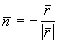The direction of the v-axis will be calculated next. The v-axis should point upwards, i.e. in the direction of the y-axis. Assuming that the "point at" position is located the origin of the world coordinate system, as the VRP is move upwards, in the y-direction, the "point at" point would move to position above the origin unless the camera system is allowed to tilt downwards as "ry" is increased. To do this the v-axis must be adjustable. The new v-axis should lie in the plane of the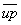and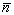vectors and perpendicular to the vector,.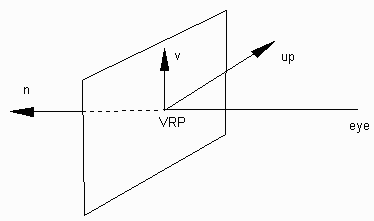The following equation will generate the necessary vector: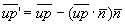The unit vector representation for the v-axis is: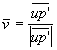The last step is to calculate the unit vector representing the u-axis. If we keep in mind that the uvn-system is a left handed system and vectorsand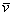are defined in terms of a right handed system, the vector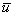is calculated using the right-hand cross product: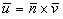## Transformation from World Coordinates to Viewing Coordinates

The camera or eye position is fixed in the viewing coordinate system. The origin of the uvn-coordinate system is located at the view reference point (VRP), described by a vector defined in world coordinates: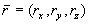The representation of a point in world coordinates is determined by the product of two transformations matrices.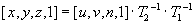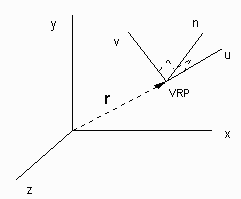Consider a point in uvn-coordinates; you must transform the point onto the equivalent world coordinate point with the understanding that the viewing system is defined in world coordinates.

In order to simplify the discussion, calculations are restricted to the uv-plane or the 2-dimensional problem. Consider point defined in xyz-coordinates as (x , y , 0) and with equivalent coordinate, (a , b, 0) in the uvn-system. Ask the question, "What transformation is required to transform the point (a , b) onto the point (x, y)?". It is possible to express the total transformation as the product of two matrices, therefore care must be taken to ensure that the transformations are performed in the proper order since the matrix representations is commutative.

The first step is to consider a move in the world system which is equivalent to moving from the origin of the uv-system onto the point (a,b). This operation is performed by moving "a units" in the direction of the u-axis and "b units" in the direction of the v-axis and is represented by the vector equation: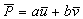whereandare unit vectors defining the uv-system coordinates. In matrix form, the transformation equation is: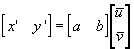Row vectorsandcan be written in component form and the problem expanded to 3-space to give the homogeneous 4-space transformation matrix.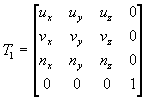It is possible, but not necessary, to show that the matrix ,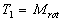is a simple rotation matrix about the origin of the world system. The geometry for the 2-D problem is summarized below: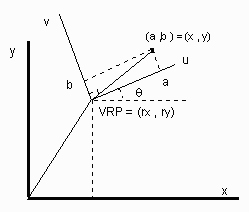In the general, the origin of the uvn-system and the origin of the xyz-system are not located at the same point. A translation of "rx units" in the direction of the x-axis, followed by a "ry units" translation in the in the direction of the y-axis is required.

This operation effectively translates the origin of the uv-system to the position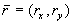as illustrated.Expanding the problem to 3-space, the translation matrix in homogeneous 4-space is written as: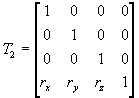The total transformation for a point with viewing coordinates,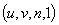to an equivalent point in the world coordinates is given by: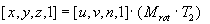The inverse transformation, world coordinates to viewing coordinate is: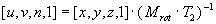It is possible to show that the inverse of a matrix product is equal to the inverse matrices multiplied in reverse order.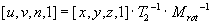Likewise, the inverse matrix for translation matrix is just the reverse translation and the inverse of the rotation matrix is the transpose of rotation matrix. It is possible to prove this property without assuming that the matrix is a rotation matrix by using a dot product. Why? The transformation from world coordinates to viewing coordinates is given by: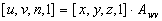where: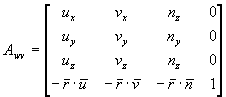Observe that the unit vectors defining the axes of the viewing system are the columns of the transformation matrix.

## The Total Transformation

The total transformation for a point in world coordinates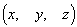to a point in viewing coordinates, assuming that the eye is positioned at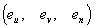, is: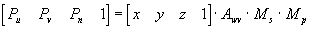The eye or COP location is defined in the uvn-system. The matrix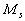takes into account that the eye need not lie on the n-axis, where: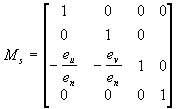The derivation for this matrix has not been presented. For the special case, the COP location is on the n-axis, i.e.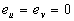, the matrixreduces to the identity matrix.

The perspective transformation matrix,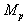is given by: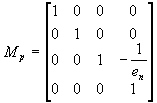The location of the eye on the n-axis is generally negative for a left-handed system. The perspective transformation moves each vertex point in three dimensions onto 2-space. The project point is drawn using: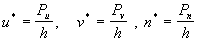n* represents a "pseudodepth" and does not have the same meaning as the other two coordinates. The project coordinates are u* and v* where n* is set to zero.Written by Ross Bradbeer, September 24, 1997